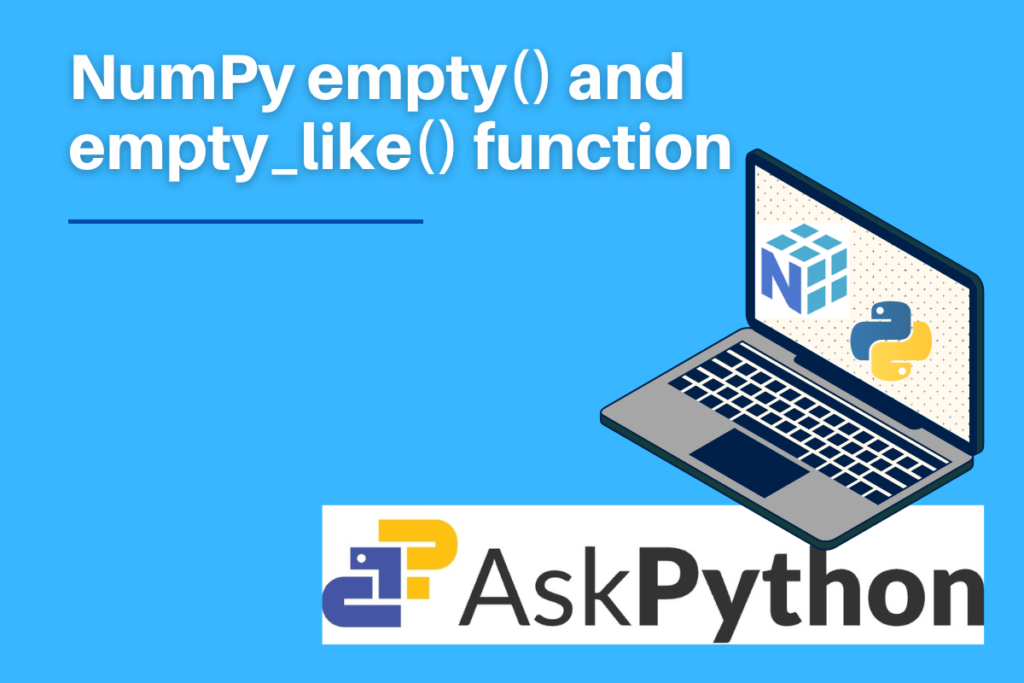# NumPy empty and empty_likeIn this article, we will try to understand the empty() function and the empty_like() function of NumPy in Python.

The Python package NumPy is used to manipulate arrays. Numerous mathematical operations can be carried out on an array with NumPy. It provides a vast library of high-level mathematical functions that work on these arrays and matrices and strong data structures that ensure efficient calculations with arrays and matrices.

In the year 2005, Travis Oliphant developed NumPy. You can use it for free because it is an open-source project.

Also read: NumPy ones_like – A Complete Guide

## What is NumPy empty?

A new array of the specified shapes and types is returned by the `numpy.empty()` function without any entries being initialized. An array of uninitialized (arbitrary) data with the specified shape, dtype, and order is the function’s result. Object arrays will begin out with a value of None.

Also remember, Since `empty` does not change the array values to zero, it can be a little bit faster than `zeros`. However, it calls for the user to manually set each value in the array, therefore it must be used with care.

## Syntax

```numpy.empty(shape, dtype=float, order='C', like=None)
```

### Parameters

• shape: int or tuple of int
• Required
• The shape of the empty array, for example:  `(4, 2)` or 4.
• dtype: data-type,
• optional
• The desired output data type for the array
• Default set to numpy.float64
• order: {‘C’, ‘F’}
• Optional
• Which order—column-major (Fortran style) or row-major (C style)—to use when storing multidimensional data in memory
• Default set to ‘C’
• like: array_like
• Optional
• To enable the creation of arrays that aren’t NumPy arrays, reference objects are provided. The outcome will be determined by an array-like passed-in as like if it complies with the array function protocol. In this instance, it makes sure that an array object is created that is compatible with the one that was provided as an argument.

Returns: a collection/array of arbitrary (uninitialized) data with the specified order, shape, and dtype. Object arrays will start out with a value of None.

## Implementation of Numpy empty()

Before implementing the function, make sure to import NumPy Package in your IDE. To import the package run the following line of code

```import numpy as np
```

### 1. Passing integer as the Shape parameter

```np.empty(4)
```

All other parameters are considered by default.

### 2. Passing Tuple as the Shape parameter

```np.empty((3,3))
```

All other parameters are considered by default.

### 3. Assigning other parameters

```np.empty((2,3), dtype=int, order='F')

np.empty((3,2), dtype=np.float16, order='C')
```

## What is NumPy empty_like?

Similar to the empty(), this function also creates an array without initializing the values. The shape and data type of the new array created is the same as that of the given (prototype) array.

The returned array is not initialized by this function; use `zeros_like` or` ones_like` in its place to do so. Compared to the routines that actually set the array values, it might be a little bit faster.

## Syntax of Numpy empty_like()

```numpy.empty_like(prototype, dtype=None, order='K', subok=True, shape=None)
```

### Parameters

• prototype: array_like
• Required
• The returning array’s properties are defined by the prototype’s shape and data type.
• dtype: data-type
• Optional
• Overrides the data type of the result.
• order: {‘C’, ‘F’, ‘A’, or ‘K’}
• Optional
• Which order – ‘C’ for C-style, ‘F’ for Fortran Style, A for ‘F’ if the prototype is Fortran contiguous, ‘C’ otherwise. ‘K’ stands for closely resembles the prototype’s layout.
• subok: bool
• Optional
• If True, the newly formed array will utilize the prototype’s sub-class type; otherwise, a base-class array will be used. Usually sets to True.
• shape: int or sequence of ints
• Optional
• Overrides the shape of the result. Will attempt to maintain order if order=’K’ and the number of dimensions remain constant; otherwise, order=’C’ is inferred.

## Implementing Numpy empty_like()

### 1. Providing only Prototype

```x = ([9,8,7],
[6,5,4],
[3,2,1])

np.empty_like(x)
```

### 2. Assigning other parameters

```y = ([1.2, 2.4, 3.6],
[2.1, 4.2, 6.3])
np.empty_like(y, dtype=int, order='K')

z =  ([2.1, 3.2, 4.1],
[4.2, 5.3, 6.1])
np.empty_like(z, order = 'C', subok = False, shape = (3,3))
```